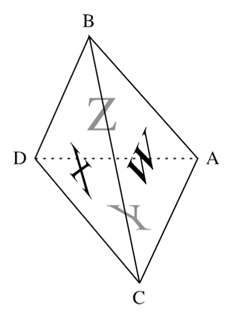## Pseudofaces of Tetrahedra

The hedronometric (“area-based”) Pythagorean Theorem for Right-Corner Tetrahedra generalizes to an unsurprising Law of Cosines:$$\displaystyle{W^2 = X^2 + Y^2 + Z^2 – 2 Y Z \cos DA – 2 Z X \cos DB – 2 X Y \cos DC}$$

for face areas $$W$$, $$X$$, $$Y$$, $$Z$$ and dihedral angles $$DA$$, $$DB$$, $$DC$$. I recently learned (while browsing Boyer’s A History of Mathematics at a Barnes & Noble) that, as early as 1803, the mathematician Carnot was aware of this result —which beats me by about 180 years— but I’m not yet sure who else may have been aware of this variation:

$$\displaystyle{W^2 + X^2 – 2 W X \cos BC = Y^2 + Z^2 – 2 Y Z \cos DA}$$

or who else, better still, may have thought the above looked too much like the trigonometric Law of Cosines not to introduce an element, say $$H$$, such that

$$\displaystyle{W^2 + X^2 – 2 W X \cos BC = H^2 = Y^2 + Z^2 – 2 Y Z \cos DA}$$

For early TeX practice in 2005, I transcribed some old journal notes recognizing $$H$$ as not just an algebraic imperative but a legitimate geometric aspect of a tetrahedron, and I dubbed the thing a “pseudoface”. While my notes —“Pseudofaces of Tetrahedra”— are somewhat clumsy and haphazard, subsequent research has indicated that this pseudoface idea actually constitutes a pretty interesting part of tetrahedral lore. I explore that lore in later papers … with (I like to think) increasingly-improved prose and TeX-ification.

Posted 17 July, 2012 by in Hedronometry